# `sklearn.metrics`.confusion_matrix¶

`sklearn.metrics.``confusion_matrix`(y_true, y_pred, labels=None, sample_weight=None)[source]

Compute confusion matrix to evaluate the accuracy of a classification

By definition a confusion matrix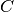is such that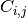is equal to the number of observations known to be in groupbut predicted to be in group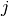.

Thus in binary classification, the count of true negatives is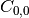, false negatives is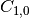, true positives is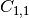and false positives is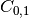.

Read more in the User Guide.

Parameters: y_true : array, shape = [n_samples] Ground truth (correct) target values. y_pred : array, shape = [n_samples] Estimated targets as returned by a classifier. labels : array, shape = [n_classes], optional List of labels to index the matrix. This may be used to reorder or select a subset of labels. If none is given, those that appear at least once in `y_true` or `y_pred` are used in sorted order. sample_weight : array-like of shape = [n_samples], optional Sample weights. C : array, shape = [n_classes, n_classes] Confusion matrix

References

Examples

```>>> from sklearn.metrics import confusion_matrix
>>> y_true = [2, 0, 2, 2, 0, 1]
>>> y_pred = [0, 0, 2, 2, 0, 2]
>>> confusion_matrix(y_true, y_pred)
array([[2, 0, 0],
[0, 0, 1],
[1, 0, 2]])
```
```>>> y_true = ["cat", "ant", "cat", "cat", "ant", "bird"]
>>> y_pred = ["ant", "ant", "cat", "cat", "ant", "cat"]
>>> confusion_matrix(y_true, y_pred, labels=["ant", "bird", "cat"])
array([[2, 0, 0],
[0, 0, 1],
[1, 0, 2]])
```

In the binary case, we can extract true positives, etc as follows:

```>>> tn, fp, fn, tp = confusion_matrix([0, 1, 0, 1], [1, 1, 1, 0]).ravel()
>>> (tn, fp, fn, tp)
(0, 2, 1, 1)
```

## Examples using `sklearn.metrics.confusion_matrix`¶Faces recognition example using eigenfaces and SVMs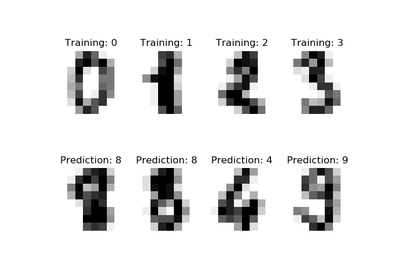Recognizing hand-written digits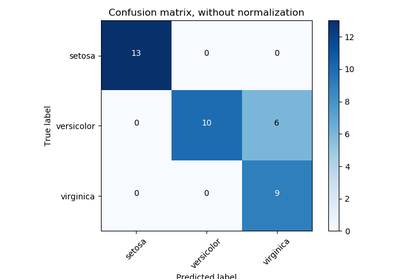Confusion matrix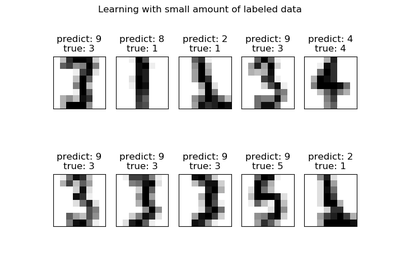Label Propagation digits: Demonstrating performance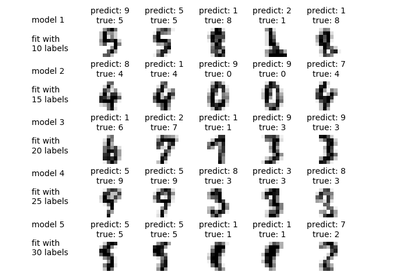Label Propagation digits active learningClassification of text documents using sparse features